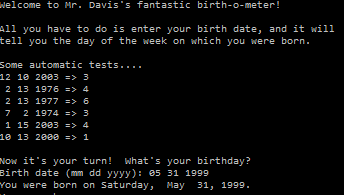# Assignemnt #96, Weekday Calculator

## Code

```/// Name: Alex Pinder
/// Period: 5
/// Program Name: Weekday Calculator
/// File Name: WeekdayCalculator.java
/// Date Complete: 6/4/16

import java.util.Scanner;

public class WeekdayCalculator
{
public static void main( String[] args )
{
Scanner keyboard = new Scanner(System.in);

System.out.println("Welcome to Mr. Davis's fantastic birth-o-meter!");
System.out.println();
System.out.println("All you have to do is enter your birth date, and it will");
System.out.println("tell you the day of the week on which you were born.");
System.out.println();
System.out.println("Some automatic tests....");
System.out.println("12 10 2003 => " + weekday(12,10,2003));
System.out.println(" 2 13 1976 => " + weekday(2,13,1976));
System.out.println(" 2 13 1977 => " + weekday(2,13,1977));
System.out.println(" 7  2 1974 => " + weekday(7,2,1974));
System.out.println(" 1 15 2003 => " + weekday(1,15,2003));
System.out.println("10 13 2000 => " + weekday(10,13,2000));
System.out.println();

System.out.print("Birth date (mm dd yyyy): ");
int mm = keyboard.nextInt();
int dd = keyboard.nextInt();
int yyyy = keyboard.nextInt();

System.out.println("You were born on " + weekday_name(weekday(mm, dd, yyyy)) + ", " + month_name(mm) + " " + dd + ", " + yyyy + ".");
System.out.println("You were born on ");
}

public static int weekday( int mm, int dd, int yyyy )
{

int yy, total;
String date = "";

yy = yyyy - 1900;
total = yy / 4;
total = total + yy;
total = total + dd;
total = total + month_offset(mm);
if (isLeap(yyyy) == true && (mm == 1 || mm == 2))
total--;
total = total % 7;

}
public static String weekday_name( int weekday )
{
String result = "";

if ( weekday == 1 )
{
result = "Sunday";
}
else if ( weekday == 2 )
{
result = " Monday ";
}
else if ( weekday == 3 )
{
result = " Tuesday ";
}
else if ( weekday == 4 )
{
result= " Wednesday";
}
else if ( weekday == 5 )
{
result = " Thursday";
}
else if ( weekday == 6 )
{
result = " Friday ";
}
else if ( weekday == 7 );
{
result = "Saturday";
}

return result;
}

public static String month_name( int n )
{
String monthName="";

if (n == 1)
{
monthName = " Jan ";
}
else if (n == 2)
{
monthName = " Feb ";
}
else if ( n == 3)
{
monthName = " Mar ";
}
else if ( n == 4 )
{
monthName = " Apr ";
}
else if ( n == 5 )
{
monthName = " May ";
}
else if ( n == 6 )
{
monthName = " June ";
}
else if ( n == 7 )
{
monthName = "Jul";
}
else if ( n == 8 )
{
monthName = " Aug ";
}
else if ( n == 9 )
{
monthName = "Sept";
}
else if ( n == 10 )
{
monthName = "Oct";
}
else if ( n == 11 )
{
monthName = "Nov";
}
else if ( n == 12 )
{
monthName = "Dec";
}

return monthName;
}
public static int month_offset(int n )
{
int monthOffset=0;

if ( n == 1 )
{
monthOffset = 1;
}
else if ( n == 2 )
{
monthOffset = 2;
}
else if ( n == 3 )
{
monthOffset = 3;
}
else if ( n == 4 )
{
monthOffset = 4;
}
else if ( n == 5 )
{
monthOffset = 5;
}
else if ( n == 6 )
{
monthOffset = 6;
}
else if ( n == 7 )
{
monthOffset = 7;
}
else if ( n == 8 )
{
monthOffset = 8;
}
else if ( n == 9 )
{
monthOffset = 9;
}
else if ( n == 10 )
{
monthOffset = 10;
}
else if ( n == 11 )
{
monthOffset = 11;
}
else if ( n == 12 )
{
monthOffset = 12;
}
return monthOffset;
}

// paste your methods from MonthName, WeekdayName, and MonthOffset here

public static boolean isLeap( int year )
{
// years which are evenly divisible by 4 are leap years,
// but years divisible by 100 are not leap years,
// though years divisible by 400 are leap years
boolean result;

if ( year%400 == 0 )
result = true;
else if ( year%100 == 0 )
result = false;
else if ( year%4 == 0 )
result = true;
else
result = false;

return result;
}
}

```

### Picture of the output